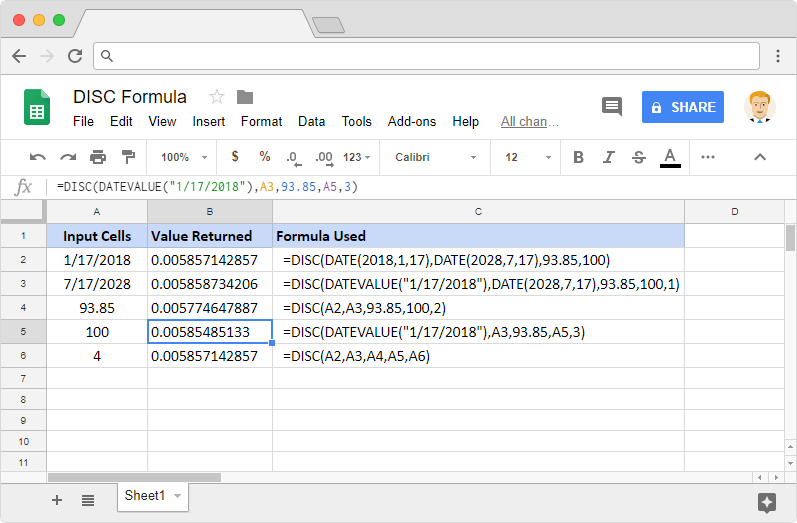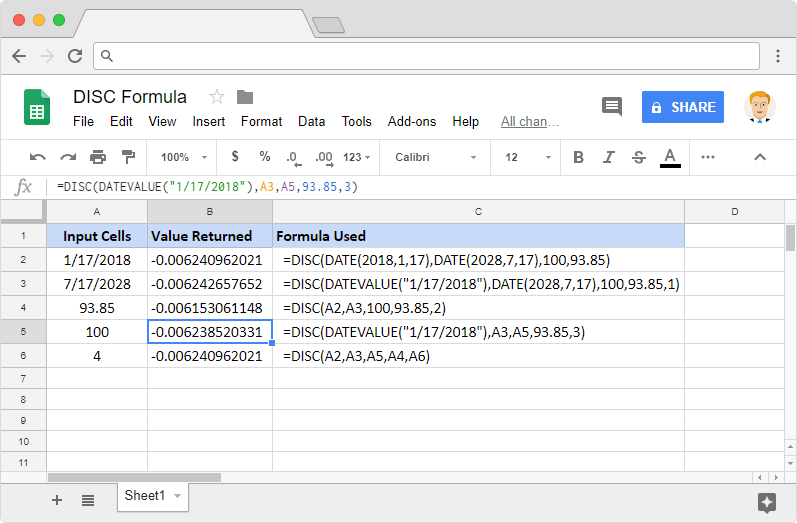# How to use the DISC formula in Google SheetsWith the DISC formula in Google Sheets, we can calculate a security’s discount rate based on its price. This is one of the myriads of Financial formulas that Google Sheets is ported with.

### Syntax

DISC(settlement, maturity, price, redemption, [day_count_convention])

• settlement – is the date after issuing the security, when it is actually delivered to the buyer.
• maturity – is the end or maturity date of the security, when the buyer can redeem it at face or par value.
• price – is the price at which the buyer bought the security for.
• redemption – is the redemption value of the security.
• day_count_convention – [OPTIONAL parameter – 0 by default ] – it is an indicator of the day count method that Google Sheets should consider. There are five different possible values for this parameter.
• 0 – assumes that there are 30 day months and 360 day years (US (NASD) 30/360). Using this value ensures there are specific adjustments to the entered dates that usually are at the end of months.
• 1 – calculates based on the actual number of days between the specified dates, and the actual number of days in the intervening years.
• 2 – calculates based on the actual number of days between the specified dates, but assumes a 360 day year.
• 3 – evaluates the DISC formula in Google Sheets based on the actual number of days between the specified dates, but assumes a 365 day year.
• 4 – very similar to the first option 0, except, it adjusts end-of-month dates according to European financial conventions.

### Usage: DISC formula in Google Sheets

Nothing helps us as much as examples do to understand a concept, so let’s use a few of them. Please see the snapshot below.Please note that we should ensure that we enter only valid dates for settlementand maturity parameters. They can be values returned from functions such as DATE, TO_DATE, or references to other date type cells. We cannot enter direct text values for dates. The other parameters can be direct numeric values or can be references to the cells holding the appropriate values.

The DISC formula can also return negative values depending upon price and redemption values. Please see the illustration below.We will notice that even if all the first four parameters are essentially the same, the output values vary. This is because of the method with which the days are counted which we indicated using the day_count_convention parameter.

### DISC formula

And there you go! Use the DISC formula in Google Sheets to calculate a security’s discount rate based on its price.

If you’d like to learn more about the various formulas of Google Sheets, why not take a look at our blog post on the PPMT formula in Google Sheets.

Alternatively, check out related blog posts below!